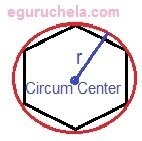# Regular Polygon Circumcircle Area Calculator

Calculates the Circumradius, Area of the polygon, Area of the circumcircle and Ratio of the area for given values.

 Enter the number of sides of the polygon (minimum 3) Enter the length of side of the polygon

 Circumradius Area of the circumcircle Area of the polygon Ratio of the area

### Formula

Circumradius (r) = l / (2 x sin( π / n ))

Area of the polygon = ( n x l2 ) / ( 4 x tan( π / n ) )

Regular Polygon Circumcircle Area = 3.14 x r2

Where,

n = Number of sides of the polygon,